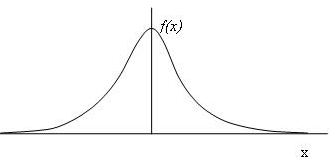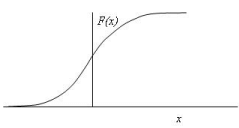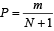# Design Flood Estimation - 2 Notes | Study Engineering Hydrology - Civil Engineering (CE)

## Civil Engineering (CE): Design Flood Estimation - 2 Notes | Study Engineering Hydrology - Civil Engineering (CE)

The document Design Flood Estimation - 2 Notes | Study Engineering Hydrology - Civil Engineering (CE) is a part of the Civil Engineering (CE) Course Engineering Hydrology.
All you need of Civil Engineering (CE) at this link: Civil Engineering (CE)

Design flood for diversion works and coffer dam

Whenever a hydraulic structure like a dam or a barrage is constructed across a river, a temporary structure called a coffer dam is built first for obstructing the river flow and the water diverted though a diversion channel or tunnel.  The Bureau of Indian Standards in its guideline IS: 10084 (Part I) -1982, “Criteria for design of diversion works - Part I: Coffer Dams” recommends the following:

“The coffer dam being a temporary structure is normally designed for a flood with frequency less than that for the design of the main structure.  The choice of a particular frequency shall be made on practical judgment keeping in view the construction period and the stage of construction of the main structure and its importance.  Accordingly, the design flood is chosen.

For seasonal cofferdams (those which are constructed every year and washed out during the flood season), and the initial construction stages of the main structure, a flood frequency of 20 years or more can be adopted.  For coffer dams to be retained for more than one season and for the advanced construction stage of the main structure, a flood of 100 years frequency may be adopted”.

Flood for cross drainage works

Cross drainage works are normally encountered in irrigation canal network system.  Generally canals flow under gravity and often are required to cross local streams and rivers. This is done by either conveying the canal water over the stream by overhead aqueducts or by passing below the stream though siphon aqueducts.  These structures are called cross drainage works and according to the Bureau of Indian Standard guidelines IS:7784 (Part I) – 1993, “Code of practice for design of cross – drainage works” the following is recommended.

“Design flood for drainage channel to be adopted for cross drainage works should depend upon the size of the canal, size of the drainage channel and location of the cross drainage.  A very long canal, crossing drainage channels in the initial reach, damage to which is likely to affect the canal supplies over a large area and for a long period, should be given proper importance.

Cross drainage structures are divided into four categories depending upon the canal discharge and drainage discharge.  Design flood to be adopted for these four categories of cross drainage structures is given as in the following table:

 Category of structure Canal discharge (m3/s) Estimated Drainage discharge Note* (m3/s) Frequency of design flood ABCD 0 - 0.50.5 - 1515 - 30>30 All discharges0 - 150>1500 - 100>1000 - 150>150 1 in 25 years1 in 50 years1 in 100 years1 in 50 years1 in 100 years1 in 100 years Note **

Notes:

*This refers to the discharge estimated on the basis of river parameters corresponding to maximum observed flood level. ** in case of very large cross drainage structures where estimated drainage discharge is above 150 m3/s and canal discharge greater than 30 m3/s, the hydrology should be examined in detail and appropriate design flood adopted, which is no case shall be less than 1 in 100 years flood

Methods for design flood computations

The criteria for choosing the design flood for various types of hydraulic structures were discussed.  For each one of these, any of the following three methods are suggested:

• Probable Maximum Flood (PMF)
• Standard Project flood (SPF)
• Flood of a specific return period

The methods for evaluating PMF and SPF fall under the hydrometeorological approach, using the unit hydrograph theory.  Flood of a given frequency (or return period) is obtained using the statistical approach, commonly known as flood frequency analysis.  In every method, adequate data for carrying out the calculations are required.  The data which are required include long term and short term rainfall and runoff values, annual flood peaks series, catchment physiographic characteristics, etc.

Within the vast areal extent of our country, it is not always possible to have observations measured on every stream.  There are a large number of such ungauged catchments in India which has to rely on synthetically generated flood formulae.  The Central Water Commission in association with the India Meteorological Department and Research Design and Standard Organization unit of the Indian Railways have classified the country into 7 zones and 26 hydro-meteorologically homogeneous sub-zones, for each one of which flood estimation guidelines have been published.  These reports contain ready to use chart and formulae for computing floods of 25, 50 and 100 year return period of ungauged basins in the respective regions.

In the subsequent section, we look into some detail about the calculations followed for the computation of

• PMF and SPF by the hydrometeorological approach.
• Evaluation of a flood of a given frequency by statistical approach.

The hydro-meteorological approach

The probable maximum flood (PMF) or the standard project flood (SPF) is estimated using the hydro-meteorological approach.  For the PMF calculations the worst possible maximum storm (PMS) pattern is estimated.  This is then applied to the unit hydrograph of the catchment to obtain the PMF.  For the calculation so the SPF, the worst observed rainfall pattern (called the Standard Project Storm or SPF) is applied to the unit hydrograph derived for the catchment.

For the estimation of the PMS or the SPS, which falls under the hydrometeorological approach, an attempt is made to analyze the causative factors responsible for the production of severe floods.  The computations mainly involve estimation of a design storm hyetograph (from past long-term rainfall data within the catchment) and derivation of the catchment response function used which can either be a lumped model or a distributed-lumped model.  In the former, a unit hydrograph is assumed to represent the entire catchment area.  In the distributed-lumped model, the catchment is divided into smaller sub-regions or sub-catchment and the unit hydrographs of each sub-region are applied together with channel routing and sometimes reservoir routing to produce the catchment response.

PMF/SPF calculation method by the hydro-meteorological approach involves the following steps:

• Data requirement for PMF/SPF studies
• Steps for evaluating PMF/SPF
• Limitation of PMF/SPF calculations

These are explained in detail in the additional section at the end of this lesson.

The statistical approach

The statistical approach for design flood estimation, otherwise also called flood frequency analysis, may be performed on the past recorded data of annual flood peak discharges either directly observed at the site or estimated by a suitable method.  Alternatively, frequency analysis may be carried out on the available record of annual rainfall events of the region.

The probability of occurrence of event (say, the maximum flood discharge observed or likely to occur in a year at a location on a river), whose magnitude is equal to or in excess of a specified magnitude X is denoted by P.  A related term, the reccurrence interval (also known as the return period) is defined as T = 1/P.  This represents the average interval between the occurrence of a flood peak of magnitude equal to or greater than X.

Flood frequency analysis studies interpret past record of events to predict the future probabilities of occurrence and estimate the magnitude of an event corresponding to a specific return period.  For the estimation of flood flows of large return periods, it is often necessary to extrapolate the magnitude outside the observed range of data.  Though a limited extrapolation to about twice the length of the record (that is, the number of years of data that is available) expected to yield reasonable accuracy, often water resources engineers are required to project much more than that.

Calculations for flood frequency

Basic to all frequency analyses, is the concept that there is a collection of data, called the ‘population’.  For flood frequency studies, this population are taken as the annual maximum flood occurring at a location on a river (called the site).  Since the river has flooded during the past years and is likely to go on flooding over the coming years (unless something exceptional like drying up of the river happens!), the recorded flood peak values which have been observed for a finite number of years are only a sample of the total population.   Here, ‘flood peak’ means the highest recorded discharge value for the river at any year. The following assumptions are generally made for the data:

• The sample is representative of the population.  Thus, it is assumed that though only a finite years’ data of peak flow has been recorded, the same type of trend was always there and would continue to be so in future.
• The data are independent.  That is, the peak flow data which has been collected are independent of each other.  Thus, the data set is assumed to be random.  In a random process, the value of the variant does not depend on previous or next values.

Flood frequency analysis starts by checking the consistency of the data and finding the presence of features such as trend, jump, etc.  Trend is the gradual shift in the sample data, either in the increasing or decreasing directions.  This may occurs due to human interference, like afforestation or deforestation of the watershed.  Jump means that one or a few of the data have exceptional values – high or low, due to certain factors, like forest fire, earthquake, landslide, etc which may change the river’s flow characteristics temporarily.

The next step is to apply a convenient probability distribution curve to fit the data set.  Here, it is assumed that yearly observed peak flow values are random numbers and which are also representative of the population, which includes all flood peak values, even these which have not been recorded or such floods which are likely to happen in future.  Each data of the set is termed as a variate, usually represented by ‘x’ and is a particular value of the entire data range ‘X’.

The probability of a variable is defined as the number of occurrences of a variate divided by the total number of occurrences, and is usually designated by ‘P’. The total probability for all variates should be equal to unity, that is,∑P =1.  Distribution of probabilities of all variates is called Probability Distribution, and is usually denoted a f(x) as shown in Figure 4.FIGURE 4. A typical probability distribution

The cumulative probability curve, F(x) is of the type as shown in Figure 5.FIGURE 5. Cumulative probability curve

The cumulative probability, designated as P(x ≤ x), represents the probability that the random variable has a value equal to or less than certain assigned value x is equal to 1 – P(x ≤ x), or P(x ≥ x).

In the context of flood frequency analysis, we may use the above concepts by assuming the recorded yearly flood peaks as the variate ‘x’.  Then, if the functions f(x) or F(x) becomes known, then it is possible to find out the probability with which certain high flood peak is likely to occur.  This idea may be used to recalculate the high flood peak that is likely to be equalled or exceeded corresponding to a given frequency (say, 1 in 100 years).

There are a number of probability distributions f (x), which has been suggested by many statisticians.  Of these, the more common are

• Normal
• Log – normal
• Pearson Type III
• Gumbel

Which one of these fits a given data set has to be checked using certain standard statistical tests. Once a particular distribution is found best, it is adopted for calculation of floods likely to occur corresponding to specific return periods.

Details of the above methods may be found in the additional section at the end of this lesson.

Plotting positions

So far we talked about extrapolation of the sample data.  However, if probability is to be assigned to a data point itself, then the ‘plotting position’ method is used.  Here, the sample data (consisting of, say, N values) is arranged in a decreasing order.  Each data (say the event X) of the ordered list is then given a rank ‘m’ starting with 1 for the highest up to N for the lowest of the order.  The probability of exceedence of X over a certain value x, that is P(X ≥ x) is given differently by different researchers, the most common of which are as given in the table below:

 SI. No Name of formula P(X ≥ x) 1 California m/N 2 Hazen (m-0.5)/N 3 Weibull m/(N+1)

Of these, the Weibull formula is most commonly used to determine the probability that is to be assigned to data sheet.

Example showing application of plotting positions:

The application of the method of plotting may be explained better with an example. Assume that the yearly peak flood flows of a hypothetical river measured at a particular location over the years 1981 to 2000 is given as in the following table. The data is to used to calculate the flood peak flow that is likely to occur once every 10 years, and once every 50 years.

 Year Peak flood  (m3/s) 19811982198319841985198619871988198919901991199219931994199519961997199819992000 700810470300440600350290330670540430320420690400360510910100

Rearranging table according to decreasing magnitude, designate a plotting position and calculate the probability of exceedence by, say, the Weibull formula shown in the following table which also gives the Return Period T (1/P).

 m Peak flood (m3/s) ProbabilityReturn periodT = 1/P years 1 910 0.048 21.000 2 810 0.095 10.500 3 700 0.143 7.000 4 690 0.190 5.250 5 670 0.238 4.200 6 600 0.286 3.500 7 540 0.333 3.000 8 510 0.381 2.625 9 470 0.429 2.333 10 440 0.476 2.100 11 430 0.524 1.909 12 420 0.571 1.750 13 400 0.619 1.615 14 360 0.667 1.500 15 350 0.714 1.400 16 330 0.762 1.313 17 320 0.810 1.235 18 300 0.857 1.167 19 290 0.905 1.105 20 100 0.952 1.050

The document Design Flood Estimation - 2 Notes | Study Engineering Hydrology - Civil Engineering (CE) is a part of the Civil Engineering (CE) Course Engineering Hydrology.
All you need of Civil Engineering (CE) at this link: Civil Engineering (CE)Use Code STAYHOME200 and get INR 200 additional OFF

## Engineering Hydrology

17 videos|22 docs|17 tests

Track your progress, build streaks, highlight & save important lessons and more!

,

,

,

,

,

,

,

,

,

,

,

,

,

,

,

,

,

,

,

,

,

;